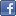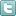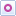EE Times-India > Amplifiers/Converters

Amplifiers/Converters# Audio pitch-shifting and the Constant-Q method

Posted: 19 Nov 2013Print Version

Keywords:DSP  pitch shifting  Short Time Fourier Transform  STFT  FFTs

In the days of audio tape, I would occasionally run across a recording that sounded a bit extraterrestrial, only to figure out it was because the tape had been running at a slightly lower or higher speed than it should have. So, I'd reach for the pitch correction knob. That knob would of course adjust two things – the pitch as well as the speed – which was usually fine, because a tape speed drift would have messed up both.

In the DSP age, we've been able to manipulate speed and pitch independently. For example, you can increase speed without changing pitch when you don't want listeners to really comprehend the terms and conditions at the end of an advertisement, or, reduce the pitch if you want to sound like a more masculine rock artist. The possibilities are huge. Let's look at one of the recent ideas in pitch shifting and what problems it addresses.

Pitch-shifting 101
If we had a nice steady-state signal (not necessarily a sine wave), pitch shifting would be easy, almost trivial. You would take the frequency spectrum, slide it up or down, then reconvert the modified spectrum to the time domain. What must one do with non-stationary music signals?

Enter, the Short Time Fourier Transform (STFT). Here, the input signal is segmented into narrow time intervals or bins (i.e., narrow enough to be considered stationary), and then a Fourier spectrum is computed for each bin. That is to say, instead of computing one FFT of a signal x[n], we window it and compute multiple FFTs, i.e, the FFTs of x[n]W[n-m], where W[n-m] is the windowing function centred at n=m. (It is the windowing function that decides the position and width of the bin.) This gives us a time varying series of FFTs, one per bin. Since each bin represents an approximately steady-state time-domain signal, its FFT can be shifted up or down as before to generate a pitch-shifted signal. Mathematically, the STFT looks like this – effectively the Fourier transform of the windowed signal x[n]W[n-m]:Sliding the window in time is tantamount to increasing the value of m. When dealing with music in real-time we can just assume we are working with the first N samples at any instant of time; so the window starts and stops with the samples at n=0 and n=N-1:The problem
The resolution of the windowing function W[] in the standard STFT is the same for all values of the frequency ω – i.e., whether it's a bass drum or the crash of cymbals represented by x[n], the bin width is the same. Further, the standard STFT has equally spaced frequencies because the exponent increases linearly with k.

Both these are terribly suboptimal because the ear's response is logarithmic, not linear. That means that if a given bin width provides just about sufficient resolution at 10kHz, it will prove woefully inadequate at 100Hz, and if it provides just enough resolution at 100Hz, it will eat precious resources computing at 10kHz where such resolution is far more than required.

The solution
To treat low and high frequencies "equally", we need to accomplish two things: (i) have bin widths that are equal in octaves, not in absolute frequencies, and (ii) space the frequencies in the spectrum logarithmically just as we do with Bode plots. So, a logarithmic bin frequency resolution is more appropriate in that it leads to a more uniform treatment of signals and a more optimum utilisation of computing power.

 Related Articles Editor's Choice
Comment on "Audio pitch-shifting and the Constan..."

Top Ranked Articles

WebinarsVisit Asia Webinars to learn about the latest in technology and get practical design tips.

Search EE Times India
Services

Go to topConnect on FacebookFollow us on TwitterFollow us on Orkut
﻿